Photovoltaic Module Recognition Based On Image ProcessingProject cycle: Mar.2019–May.2020

1.Abstract

This project uses image processing technology to complete the recognition of photovoltaic (PV) strings and PV modules, foreign object occlusion, module damage, and the entire implementation process (as shown in Figure 1-1): first, the UAV is used to collect PV images, then identify the PV module strings in each image, then extract the PV modules in each PV string, and finally identify the abnormalities in each PV module, thus realizing the recognition and localization of PV module abnormalities in the images.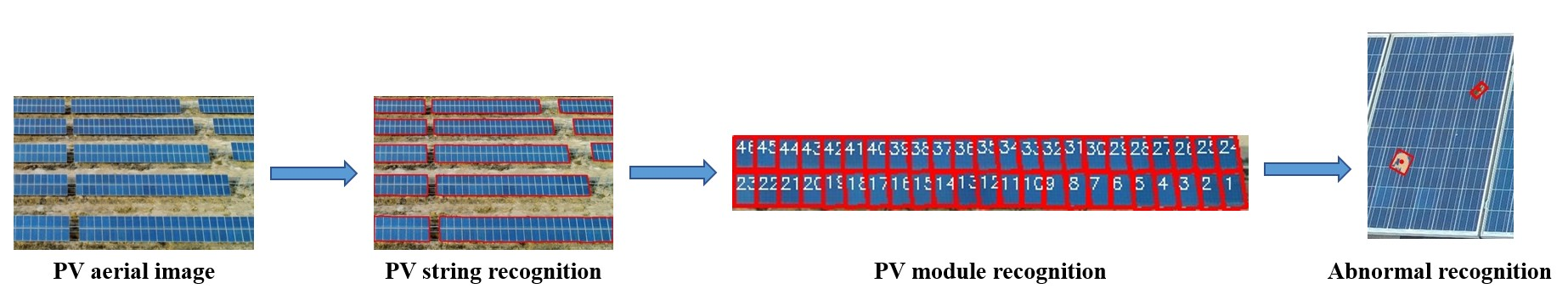Figure 1-1: The overall implementation process

For PV string recognition, the biggest difference between the PV string and the rest of the image is the color. the PV string presents a large blue area. Therefore, the key to recognize the PV string is the color-based image segmentation technique. After the PV string recognition is completed, the K-means++ clustering algorithm is used to achieve the recognition of PV modules by combining the image color and grayscale features.

For anomaly recognition such as foreign object shielding and module damage, the background plate, grid lines and frame are first eliminated using HSV space, morphological processing, Canny operator and probabilistic Hough transform. Then, the anomalous contours are extracted and filtered using contour extraction and minimum outer rectangle.

The specific implementation steps will be described in detail in the following chapters.

2.PV string recognition

The biggest difference between the PV string and other parts in the image is the color difference. Therefore, the key to recognize the PV string is the color-based image segmentation technology. The implementation process is shown in Figure 2-1: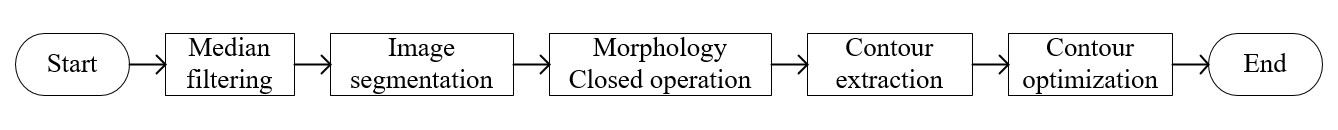Figure 2-1: The implementation process of PV string recognition

2.1.Median filtering

In the process of image acquisition and transmission, UAV shooting jitter and data transmission will cause some noise information in the image, which will affect the recognition of PV string. Therefore, it is necessary to filter the image to reduce noise interference and retain the real information of the image.

Median filtering is to replace the gray value of the original pixel with the median value of the gray value in the neighborhood of the pixel, which can effectively suppress the impulse noise and salt and pepper noise, so as to achieve the purpose of smoothing the PV image.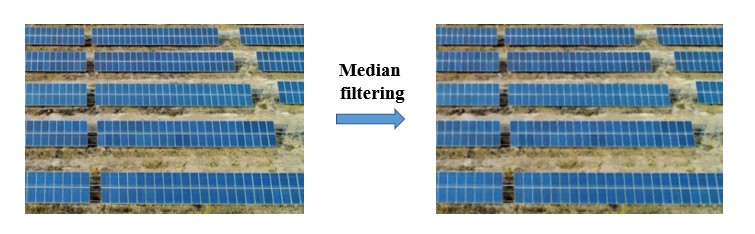Figure 2-2: Median filtering

2.2.Image segmentation

The blue characteristic of PV string is used for image segmentation, and the image is transformed into HSV model, and HSV parameters include hue (H), saturation (s) and brightness (V). Each color in the color space has a certain value range. According to the value range of blue in HSV color model, the PV string can be identified and binarized. The result is shown in Figure 2-3: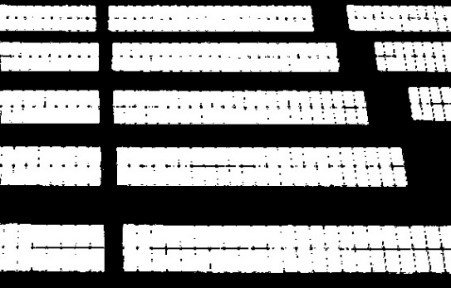Figure 2-3: Image segmentation based on HSV

2.3.Morphological closed operation

Morphological operation is an image processing algorithm based on mathematical topology. It can eliminate interference and highlight some specific areas by combining a variety of operations. Morphological close operation is used to eliminate the black holes in the white area. The result is shown in Figure 2-4: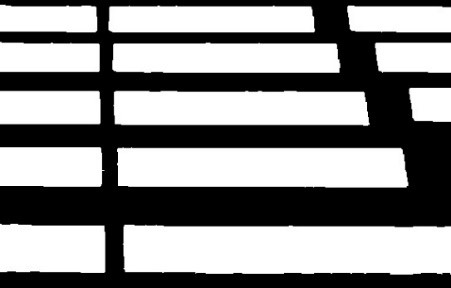Figure 2-4: Morphological closed operation

2.4.Contour extraction

Eight neighborhood traversal algorithm is used to extract the contour information of PV string.

Firstly, the condition that the contour should satisfy is defined: if the point belongs to white pixel and there is more than one black pixel in its eight neighborhood, it is considered as contour point, otherwise it is not contour point; Then, eight neighborhood is used to traverse all the pixels in the binary image to find out all the contour points that meet the conditions, and the specific location information of the points is recorded; Finally, the set of position information of all contour points is obtained, and the contour set is displayed on the original image to get the final recognition result of PV string (as shown in Figure 2-5).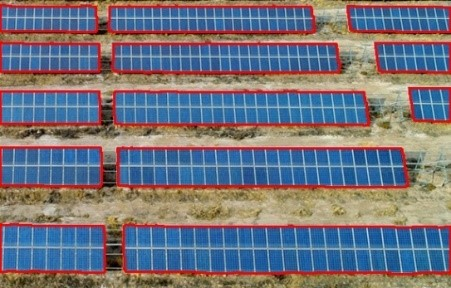Figure 2-5: Contour extraction

2.5.Contour optimization

After the contour extraction, the contour of PV string is basically clear, but there are some jitters and false recognition in the contour boundary. In this paper, the minimum external rectangle and contour area are used to optimize the contour recognition results. Firstly, the smallest external rectangle in all contours is recognized, and then the area of all external rectangles is calculated. According to the area characteristics of PV string, the smaller contours are excluded, and finally the contours of all PV strings are recognized and numbered. The processing results are shown in Figure 2-6: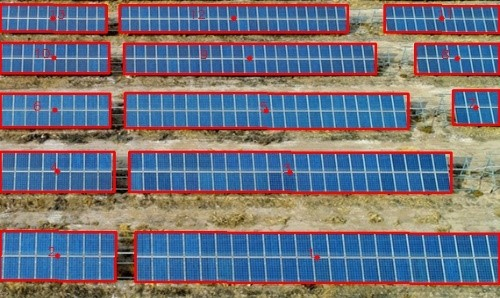Figure 2-6: Contour optimization

3 PV module recognition

After recognizing the PV string, the contour and position of each PV module are recognized, The implementation process is shown in Figure 3-1: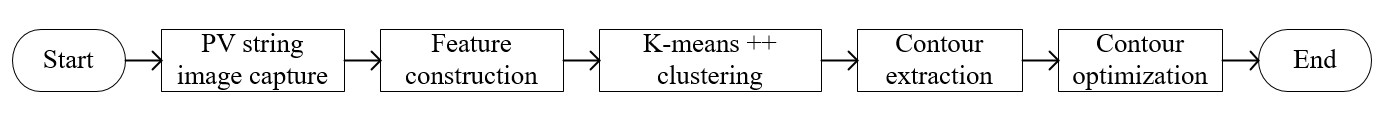Figure 3-1: The implementation process of PV module recognition

3.1.PV string image capture

The position of each PV string is captured, and the captured image of single PV string is obtained, as shown in Figure 3-2: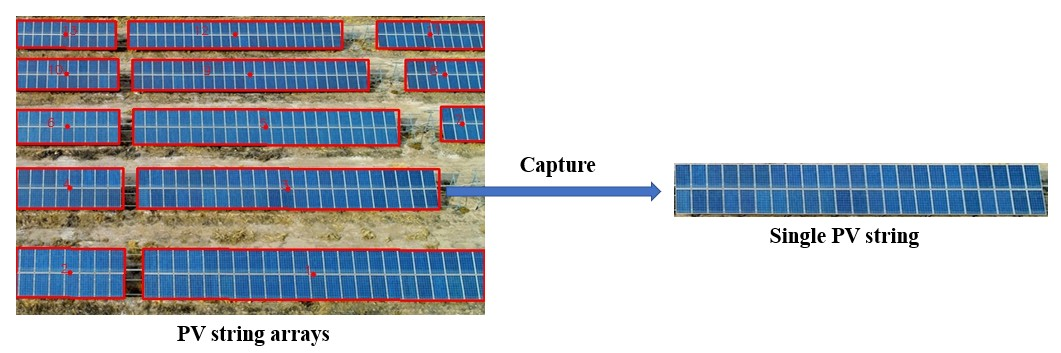Figure 3-2: PV string image capture

3.2.Feature construction

Gray features are extracted by graying the image, and color features are extracted by transforming the original image into HSV space. Finally, the feature vector is 4 dimensions, which are h, s, V and gray value, as shown in formula (3-1): $$x={x_i|i=1,2,3,4} \tag{3-1}$$

3.3.K-means++ image segmentation

Firstly, cluster centers are obtained by K-means++. Due to the special image of PV module, the pixels in the image are mainly distributed on the frame and PV panel, so the number of clusters is set to 2.

Secondly, the Euclidean distance is used to divide the pixel and the cluster center, such as pixel $x_i=(x_{i_1},x_{i_2},x_{i_3},x_{i_4})$, cluster center pixel $x_j=(x_{j_1},x_{j_2},x_{j_3},x_{j_4})$, the Euclidean distance $d(x_i,y_j)$ between them as shown in formula (3-2): $$d(x_i,y_j)=\sqrt{\sum_{n=1}^4(x_{i_n}-x_{j_n})^2} \tag{3-2}$$

Then, the sum of error squares E of all data is calculated, and the convergence of E is judged. If not, the new clustering center is used to calculate E.The calculation formula is shown in formula (3-3): $$E=\sum_{i=1}^K\sum_{x_j\in{S_i}}(x_j-m_i)^2\tag{3-3}$$ Where K is the number of clusters, $S_i$ is the number of clusters is the data set of class $i$, and $x_j$ belongs to $S_i$, $m_i$ means $S_i$ mean value.

Finally, the clustering center is no longer changed, and the clustering result of PV module and the image segmentation result is shown in Figure 3-3: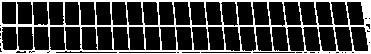Figure 3-3: K-means++ image segmentation

3.4.Contour optimization

The minimum external rectangle algorithm is used to number the contour of all PV modules. The processing results are shown in Figure 3-4: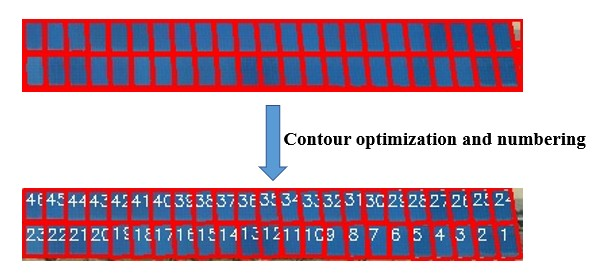Figure 3-4: Contour optimization and numbering

4.Abnormal recognition

PV modules are placed in outdoor environment all year round, often affected by wind, rain, sun and other environment, there will be some abnormal conditions, such as damage, bird dung shielding and dust stains. If these abnormal conditions can not be found in time, it will lead to photovoltaic hot spot effect and even short-circuit burning, leading to fire.

After the recognition of PV string and PV module, this chapter will detect the possible abnormalities on each PV module, and the processing flow is shown in Figure 4-1:Figure 4-1: The processing flow of abnormal recognition

4.1.Background and grid line elimination based on morphology

Firstly, the PV module image is transformed into HSV image format, and the blue background plate is extracted according to the HSV color model. Then, the blue background plate is excluded from the image to obtain the binary image, as shown in Figure 4-2 (b). Finally, the morphological close operation is used to eliminate the grid line interference in the binary image, as shown in Figure 4-2 (c):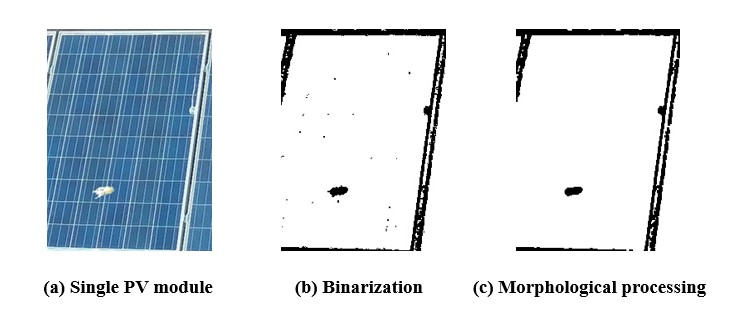Figure 4-2: Background and grid line elimination

4.2.Frame elimination based on probabilistic Hough transform

The steps are as follows:

(1) The pixels of the edge image obtained by Canny algorithm are mapped to the Hough space of polar parameters, and the corresponding curves of each edge point are obtained;
(2) These curves will form intersections in Hough space. When the number of curves corresponding to an intersection reaches the set minimum threshold, the line L in the Y-X image space coordinates corresponding to the intersection is found as a possible result;
(3) The pixel points on the line L in the border edge image are connected into line segments, and then all these points are deleted. Only the starting and ending points of the line segments are recorded, and the line segments larger than the minimum length are selected as the results;
(4) Repeat the above steps (1) (2) (3) until all the lines meeting the conditions are found;

The border line obtained by the probability Hough transform is shown in Figure 4-3(a), and it is fused with the binary image of eliminating the background plate and grid line. The border line area is set as white pixels, and the border interference is basically eliminated. The result is shown in Figure 4-3(b):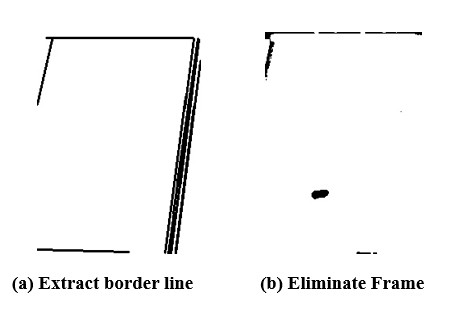Figure 4-3: Frame elimination

4.3.Abnormal area recognition

After eliminating the interference of background plate, grid line and frame, the occluded or damaged contour is exposed. Then the contour is extracted by eight neighborhood traversal algorithm, and the minimum circumscribed rectangle of the contour is calculated as the filtering condition, and the position information of the abnormal area is obtained, the result are shown in Figure 4-4: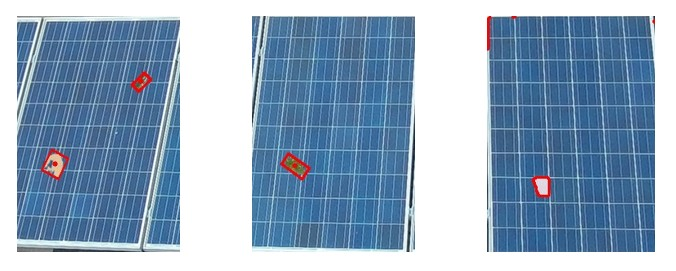Figure 4-4: Abnormal area recognition

5.Achievements

5.1.Complete the master’s thesis

According to the content of the project, further research on the related image processing technology, I completed my master’s thesis.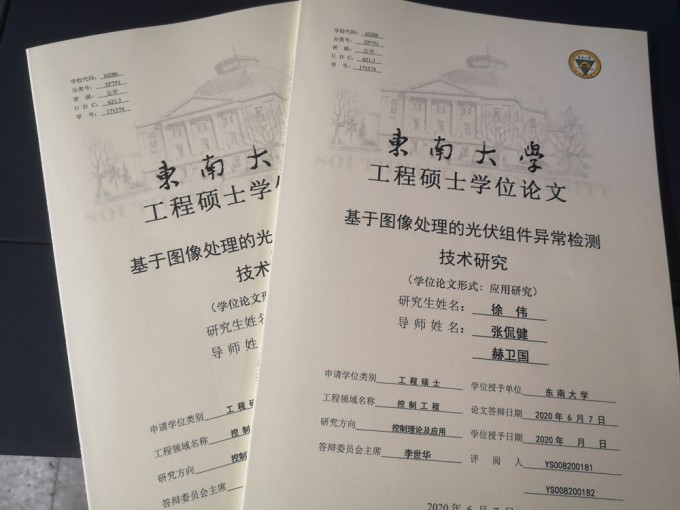Figure 5-1: Master's thesisXu Wei
Software Engineer

The charm of technology is the perfect combination of hardware and software.